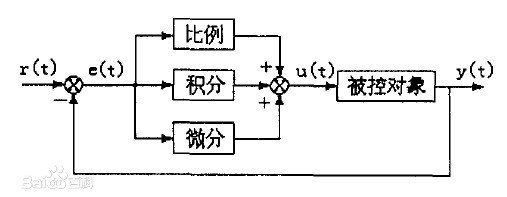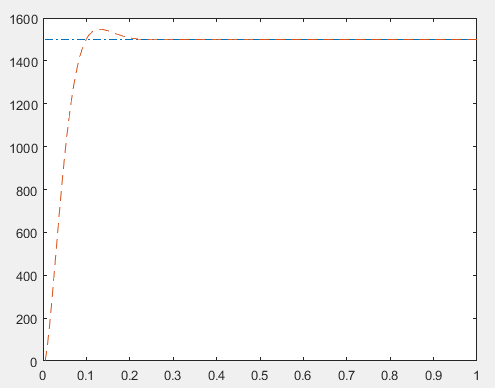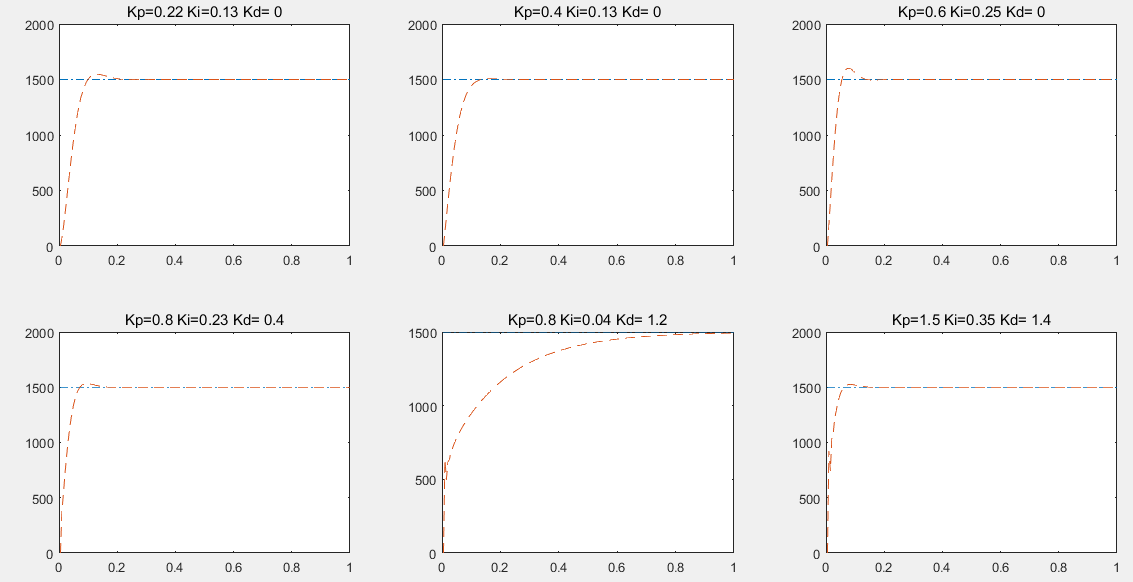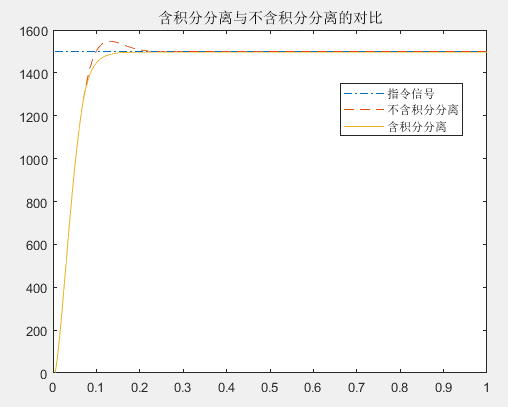• 这是基于matlab的pid控制方式，可以用matlab设计pid控制器，更加简洁优化
• 基于MATLABPID控制器设计.rar 基于MATLABPID控制器设计.rar 基于MATLABPID控制器设计.rar基于MATLABPID控制器设计.rar
• 基于 MATLABPID 控制器设计 基于 MATLABPID 控制器设计PID 控制简介 PID 控制是最早发展起来的经典控制策略 , 是用于过程控制最有效的 策略之一由于其原理简单技术成在实际应用中较易于整定 , 在工业 控制...
• 基介绍了基于Ziegler- Nichols整定方法的PID 控制器设计, 给出了基于MATLAB和Simulink的实现方法和仿真。仿真结果表明, 此算法设计PID 控制器有良好的性能指标
• 023 文章编号:1000 －2243( 2011) 06 －0898 －06 运用 Matlab 实现 PID 控制器的设计 郑剑翔 ( 福州大学现代教育技术中心，福建 福州 350108) 摘要: 传统的 PID 控制器设计中的参数调试耗时费力且调节不当还可能...
第 39 卷 第 6 期 2011 年 12 月 福州大学学报( 自然科学版) Journal of Fuzhou University( Natural Science Edition) Vol． 39 No． 6 Dec． 2011 DOI: CNKI:35 －1117/N． 20111220． 0957． 023 文章编号:1000 －2243( 2011) 06 －0898 －06 运用 Matlab 实现 PID 控制器的设计 郑剑翔 ( 福州大学现代教育技术中心，福建 福州 350108) 摘要: 传统的 PID 控制器设计中的参数调试耗时费力且调节不当还可能损坏机器． 使用 2009b 版 Matlab 软件新增的 PID 控制模块，可方便快捷地完成PID 调试工作． 以设计液压式旋切机控制器为例，分析使用 Matlab 的PID 控制模块、定点工具以及嵌入式实时代码工具完成 PID 控制器的设计，包括对象建模、离散化和定点运算处理等工作． 关键词: PID 控制; Matlab; 无卡轴旋切机; 液压 中图分类号: TP271． 2 文献标识码: A An implementation method for PID controller design by Matlab ZHENG Jian － xiang ( Modern Education Technology Center of Fuzhou University，Fuzhou，Fujian 350108，China) Abstract: Parameters adjustment is a complicated and time － consuming work in traditional PID con-troller design usually，inappropriate adjustment can cause hardware damage． By using newly added PID controller module in 2009b version of Matlab software，PID adjustments will become more conven-ience and faster． It can become easily to do PID controller design，including user’s plant modling，discrete － time implementation and fixed － point scaling in the design． Using a controller design proce-dure of hydraulic veneer lathe as example，this paper introduces how to use PID control module，fixed － piont toolbox，and embedded real － time coder of Matlab to design a PID controller easily． Keywords: PID control; Matlab; veneer peeling lathe; hydraulic pressure 0 引言 调试 PID 控制器需要确定三个参数值: 比例、积分和微分的增益． 要安全系统地找到能保证控制系统最佳运行的一组参数是一件复杂的工作［1］． 用传统的方法调试，
展开全文• 基于MatlabPID参数最优化设计 基于MatlabPID参数最优化设计
• 基于MATLABPID_控制器设计，较为详细的一个设计过程
• 基于 MATLABPID 控制器设计PID 控制简介 PID 控制是最早发展起来的经典控制策略, 是用于过程控制最有效的策 略之一由于其原理简单技术成在实际应用中较易于整定, 在工业控 制中得到了广泛的应用它最大的优点...
• MATLAB 论文 -- 基于控制系统的 PID 调节 基于 MATLABPID 控制器 摘要本论文主要研究 PID 控制器 PID 控制是迄今为止最通用的控制方法 大多数反馈回路用该方法或其较小的变形来控制 PID 控制器亦称调节器及 其...
• 在直接数字域设计中，我们常常需要用到PID算法，而PID算法投入单片机使用时，往往需要硬件的支持，在调试时非常麻烦。本文通过Matlab仿真的手段实现PID，方便了开发者对系统的设计和实时调试。
文章目录0.符号说明1.如何根据连续系统建立差分方程1.1.获取连续系统的传递函数1.2.获取离散系统的传递函数1.3.转换为差分方程2.基本PID控制原理3.比较PID输出，分析参数产生的影响4.改进PID算法（遇限削弱积分法）5.simulink仿真
0.符号说明

y(k)——系统响应输出的离散值
u(k)——数字PID控制输出的离散值
r(k)——期望输出的离散值（事先已知），在本例中为常数（即阶跃输入）
e(k)——e(k)=r(k)-y(k)，为期望值-实际值，是单位负反馈的误差比较信号注：图片来源于百度百科

1.如何根据连续系统建立差分方程
1.1.获取连续系统的传递函数
线性定常系统的控制中，PID是个非常常见的控制方式，如果可以通过Matlab仿真出PID的控制效果图，那么对系统设计时的实时调试将会容易得多。在这里我们将会以一个利用系统辨识参数的PID设计为为例展示Matlab仿真PID的过程。
首先需要对一个未知的系统的参数进行辨识，以延迟环节可以忽略不计的电机调速系统为例。将时间戳导入xdata向量，对应的时刻转速导入ydata向量，进行系统辨识
链接：Matlab的系统辨识
我们就以上文链接中辨识的系统传递函数为例：
$G(s)=\frac{0.998}{0.021s+1}$因此通过tf函数建立系统结构体如下：
sys=tf(0.998,[0.021,1]);   %建立被控对象传递函数，即式4.1

1.2.获取离散系统的传递函数
由于是数字PID仿真，我们需要选取一个采样时间，本案例选用的是0.005s（注意，采样周期应该小于系统纯滞后时间的0.1倍）。在对其进行数字PID控制前，我们需要将这个系统离散化：
ts=0.005;  %采样时间=0.005s
dsys=c2d(sys,ts,'z');      %离散化

dsys即我们根据采样周期离散化的Z变换系统。首先我们需要提取这个Z变化d那系统的参数方便后面的计算：
[num,den]=tfdata(dsys,'v');%'v'代表强制以向量的格式（默认为元胞数组）输出num和den

1.3.转换为差分方程
求解出的Z变换表达式为$dsys=\frac{num(1)\cdot z +num(2)}{den(1)\cdot z+den(2)}=\frac{0.2114}{z-0.7881}$
在PID仿真的过程中我们需要求解出时域表达式 ，因此需要借助差分方程解决，对于以下的Z变换：
\begin{equation}
Y(z)=dsys\cdot U(z)=\frac{num(2)}{den(1)\cdot z+den(2)}\cdot U(z)
\label{eq:Sample1}
\end{equation}
\begin{equation}
zY(z)+den(2)Y(z)=num(1)zU(z)+num(2)U(z)
\label{eq:Sample2}
\end{equation}
对上式进行反Z变换，可以得到以下的差分方程：
\begin{equation}
y(k+1)+den(2)y(k)=num(1)u(k+1)+num(2)u(k)
\label{eq:Sample3}
\end{equation}
\begin{equation}
y(k+1)=-den(2)y(k)+num(1)u(k+1)+num(2)u(k)
\label{eq:Sample4}
\end{equation}
位置型PID仿真时实际上可以不需要保存前一个数据(u(k)和y(k))，增量型PID必须要保存前一个数据。这里我们使用了位置型PID，但仍然利用$u_1$和$y_1$保存了上一个数据，仅仅是为了演示这一过程。\begin{equation}
y(k+1)=-den(2)y(k)+num(1)u(k+1)+num(2)u(k)
\end{equation}
可以转换为下面的式子：
\begin{equation}
y(k)=-den(2)y_1+num(1)u(k)+num(2)u_1
\label{eq:Sample5}
\end{equation}
我们的差分方程就这样建立完毕。注意，此差分方程仅仅是描述系统模型的运算规律的，和我们的控制无关。因此是y(k)和u(k)的映射关系。我们下面的控制则是利用负反馈信号e(k)导出u(k)的输出，求解的是控制器u(k)的序列值。
2.基本PID控制原理
以位置型PID控制为例。将连续的PID控制转换为数字式时，微分环节被用差分代替，积分环节被累加和代替，比例环节则保持不变。差分的实现非常简单，只需要用$e(k+1)-e(k)$即$e(k)-e_1$等效即可。积分的实现在每一次运算的后面都累加原来的误差，即Ee=Ee+e_1;即可。PID的控制器输出$u(k)=Kp\cdot e(k)+Kd\cdot (e(k)-e_1)+Ki\cdot Ee$
PID控制器构造完毕，我们需要通过r(k)和y(k)得到e(k)，再通过e(k)得出u(k)，进而再求解出y(k)，再结合r(k)求解出e(k),…以此循环，求解出离散的响应点。
详细的代码如下：
ts=0.005;  %采样时间=0.005s
sys=tf(0.998,[0.021,1]);   %建立被控对象传递函数，即式4.1
dsys=c2d(sys,ts,'z');      %离散化
[num,den]=tfdata(dsys,'v');   %
e_1=0;      %前一时刻的偏差
Ee=0;       %累积偏差
u_1=0.0;    %前一时刻的控制量
y_1=0;       %前一时刻的输出
%PID参数
kp=0.22;
ki=0.13;
kd=0;
u=zeros(1,1000);%预先分配内存
time=zeros(1,1000);%时刻点（设定1000个）
for k=1:1:1000
time(k)=k*ts;   %时间参数
r(k)=1500;      %期望值
y(k)=-1*den(2)*y_1+num(2)*u_1+num(1)*u(k);%系统响应输出序列
e(k)=r(k)-y(k);   %误差信号
u(k)=kp*e(k)+ki*Ee+kd*(e(k)-e_1); %系统PID控制器输出序列
Ee=Ee+e(k);    %误差的累加和
u_1=u(k);    	%前一个的控制器输出值
y_1=y(k);    	%前一个的系统响应输出值
e_1=e(k);		%前一个误差信号的值
end
%（仅绘制过渡过程的曲线，x坐标限制为[0,1]）
p1=plot(time,r,'-.');xlim([0,1]);hold on;%指令信号的曲线（即期望输入）
p2=plot(time,y,'--');xlim([0,1]);%不含积分分离的PID曲线
hold on;

输出的PID控制曲线如下：3.比较PID输出，分析参数产生的影响
一个基本的PID就完成了。下面如果我们想要知道修改PID的三个参数kp,ki,kd会带来什么效果，只需要在程序中修改即可。为了方便起见，我们建立一个PID的数组，kp,ki,kd每次都取数组的一个值，然后设定一个大循环开始循环仿真。再利用subplot输出子图的方式将所有的PID效果都输出到一个图进行对比。该代码根据上述代码修改已经很容易，PID比较图的代码如下：
close all
PID=[0.22,0.13,0;
0.4,0.13,0;
0.4,0.25,0;
0.8,0.23,0.4;
0.8,0.2,1;
0.7,0.2,0.9];%初始化PID参数
for pid=1:1:6
ts=0.005;  %采样时间=0.005s
sys=tf(0.998,[0.021,1]);   %建立被控对象传递函数，即式4.1
dsys=c2d(sys,ts,'z');      %离散化
[num,den]=tfdata(dsys,'v');   %
e_1=0;      %前一时刻的偏差
Ee=0;       %累积偏差
u_1=0.0;    %前一时刻的控制量
y_1=0;       %前一时刻的输出
%PID参数
kp=PID(pid,1);
ki=PID(pid,2);
kd=PID(pid,3);
u=zeros(1,1000);
time=zeros(1,1000);
for k=1:1:1000
time(k)=k*ts;   %时间参数
r(k)=1500;      %给定量
y(k)=-1*den(2)*y_1+num(2)*u_1+num(1)*u(k);
e(k)=r(k)-y(k);   %偏差
u(k)=kp*e(k)+ki*Ee+kd*(e(k)-e_1);
Ee=Ee+e(k);
u_1=u(k);
y_1=y(k);
e_1=e(k);
end
subplot(2,3,pid);
p1=plot(time,r,'-.');xlim([0,1]);hold on;
p2=plot(time,y,'--');xlim([0,1]);
title(['Kp=',num2str(kp),' Ki=',num2str(ki),' Kd= ',num2str(kd)]);
hold on;
end

输出的子图矩阵如下：可以发现，修改Kp会造成上升时间的缩短，但是有可能也会带来较大的超调。积分的增加是一个严重的滞后环节，会减小相位裕度，也会带来超调（超调量并不是绝对的，相对于较小的Kp可能会产生较大的超调，而Kp较大时超调会减小（例如第一行的1图和2图的对比））。然而积分的引入也是必要的，否则将会很长时间无法削弱误差e(k)（例如第二行第二个图）。微分的引入相当于一个超前校正，会减少超调，但是过渡的微分很可能会造成尾部振荡，系统逐渐变得不稳定。因此微分和积分之间需要一个平衡，当满足这个平衡的时候，系统几乎没有振荡，同时响应速度也较快。（第一行的图3是积分过多，产生超调，第二行的图1和图3就比较理想）
综合上述，PID的调节经验可以归结为以下几点：

Kp较小时，系统对微分和积分环节的引入较为敏感，积分会引起超调，微分可能会引起振荡，而振荡剧烈的时候超铁也会增加。
Kp增大时，积分环节由于滞后产生的超调逐渐减小，此时如果想要继续减少超调可以适当引入微分环节。继续增大Kp系统可能会不太稳定，因此在增加Kp的同时引入Kd减小超调，可以保证在Kp不是很大的情况下也能取得较好的稳态特性和动态性能。
Kp较小时，积分环节不宜过大，Kp较大时积分环节也不宜过小（否则调节时间会非常地长），在下面这个例子中我们还会介绍到，当使用分段PID，在恰当的条件下分离积分，可以取得更好的控制效果。原因在于在稳态误差即将满足要求时，消除了系统的滞后。因此系统超调会明显减少。本例中采样的抗积分饱和的方法是遇限削弱积分法。

4.改进PID算法（遇限削弱积分法）
遇限削弱积分法的原理是
当$u(k)>u_{max}$时，若e(k)>0即输出值还未到达指定值，则认为积分会带来滞后，不再积分。
当$u(k)<0$时，若e(k)<0即输出值超过了指定值，则认为积分会带来滞后，不再积分。
在本案例中认为$u_{max}=r(k)$
改进PID算法如下（需要些两个循环，当然也可以用一个循环，将其中的PID设为一个子过程调用）：
close all
ts=0.005;  %采样时间=0.005s
sys=tf(0.998,[0.021,1]);   %建立被控对象传递函数，即式4.1
dsys=c2d(sys,ts,'z');      %离散化
[num,den]=tfdata(dsys,'v');   %
e_1=0;      %前一时刻的偏差
Ee=0;       %累积偏差
u_1=0.0;    %前一时刻的控制量
y_1=0;       %前一时刻的输出
%PID参数
kp=0.22;
ki=0.13;
kd=0;
u=zeros(1,1000);
time=zeros(1,1000);
for k=1:1:1000
time(k)=k*ts;   %时间参数
r(k)=1500;      %给定量
y(k)=-1*den(2)*y_1+num(2)*u_1+num(1)*u(k);
e(k)=r(k)-y(k);   %偏差
u(k)=kp*e(k)+ki*Ee+kd*(e(k)-e_1);
Ee=Ee+e(k);
u_1=u(k);
y_1=y(k);
e_1=e(k);
end
p1=plot(time,r,'-.');xlim([0,1]);hold on;
p2=plot(time,y,'--');xlim([0,1]);
hold on;
a=1;%控制积分分离的二值数
e_1=0;Ee=0;u_1=0.0;y_1=0;%重新初始化
for k=1:1:1000
time(k)=k*ts;   %时间参数
r(k)=1500;      %给定量
y(k)=-1*den(2)*y_1+num(2)*u_1;
e(k)=r(k)-y(k);   %偏差
u(k)=kp*e(k)+ki*Ee+kd*(e(k)-e_1);
if ((u(k)>r(k)) && (e(k)>0))||((u(k)<0) && (e(k)<0))
a=0;
else
a=1;
end
Ee=Ee+a*e(k);
u_1=u(k);
y_1=y(k);
e_1=e(k);
end
p3=plot(time,y,'-');xlim([0,1]);
title('含积分分离与不含积分分离的对比');
legend([p1,p2,p3],'指令信号','不含积分分离','含积分分离');

输出的曲线对比图如下：可以发现，系统的超调量明显减少了，调节时间也减少了一点。原因在于我们采用了分段PID的手段，既消除了稳态误差还削弱了积分环节带来的滞后影响。
需要的模块名称（不区分大小写）如下：

gain（参数分别为0.22和0.13/0.005）
sum（参数分别为"|±"和"|++"）
integrator
scope

以表中第一组PI参数为例：得到的示波器曲线如下：希望本文对您有帮助，谢谢阅读。


展开全文• 基于MATLAB模糊自适应PID 控制器的设计The fuzzy PID controller and‘its simulation Abstract：This paper discusses the design of fuzzy PID controller and he method of realization using MATLAB software．...
基于MATLAB模糊自适应PID 控制器的设计The fuzzy PID controller and‘its simulation Abstract：This paper discusses the design of fuzzy PID controller and he method of realization using MATLAB software．The result of simulation in MATLAB／Simulink indicates that the controlle r evidently improves the dynamic property of control system．The controlle r is easily realized and applied in engineering．First, let's take a look at how the PID controller works in variable (e) represents the tracking error, the difference between the desired input value (R) and the actual output (Y). This error signal (e) will be sent to the PID controller, and the controller computes both the derivative and the integral of this error signal. The signal (u) just past the controller is now equal to the proportional gain (Kp) times the magnitude of the error plus the integral gain (Ki) times the integral of the error plus the derivative gain (Kd) times the derivative
展开全文• 基 于 M A T L A B 的 P I D 控 制 器 设 计 一 PID 控制简介 PID 控制是最早发展起来的经典控制策略 , 是用于过程控制最有效的策略之 一由于其原理简单技术成在实际应用中较易于整定 , 在工业控制中得到了广 泛的...
• 本文档为纯代码。在MATLAB中，设定一个不满足要求的系统，设计PID校正该系统，使其满足需求。校正代码中部分带%段落需要去掉%
• 先进PID设计Matlab程序设计，不错的资源，和大家分享！
• 基于MATLAB的模糊PID控制器的设计，使用简单，易上手。
• matlab开发-优化PID控制器设计接口。optimpid是一个基于matlab的接口，可以用来优化PID参数。
• 2020江苏省电子设计竞赛C题（省一等奖）的PID算法仿真，便于基于STM32硬件实现PID控制算法，基于matlab实现PID算法仿真，结果准确可靠，实现简单
• matlab实验十控制系统的pid校正设计及仿真.doc 实验十 控制系统的 PID 校正设计及仿真 一、实验目的 1．学会用 MATLAB 对系统进行仿真； 2．应用频率综合法对系统进行 PID 校正综合。 二、设计原理与步骤 1．设计...matlab实验十控制系统的pid校正设计及仿真.doc 实验十 控制系统的 PID 校正设计及仿真 一、实验目的 1．学会用 MATLAB 对系统进行仿真； 2．应用频率综合法对系统进行 PID 校正综合。 二、设计原理与步骤 1．设计原理超前校正的主要作用是增加相角裕量，改善系统的动态响应特性。滞后校正的作用是 改善系统的静态特性，两种校正结合起来就能同时改善系统的动态和静态特性。滞后超前 校正(亦称 PID 校正)综合了前两种校正的功能。 滞后超前校正(亦称 PID 校正)的传递函数为：) ( , ) 1 ( ) 1 )( 1 ( ) 1 )( 1 ( ) ( 1 2 1 2 1 2 T T S T S T S T S T S G C              它相当于一个滞后校正与一个超前校正相串联，其对数频率特性如 图 10-1 所示： 2．设计步骤 基于频率法综合滞后- 超前校正的步骤是： (1)根据静态指标要求，确定开环比例系数 K ，并按已确定的 K 画出系统固有部分的 Bode 图；(2)根据动态指标要求确定 ，检查系统固有部分在 的对数幅频特性的斜率是否为- c  c 2，如果是，求出 点的相角; c (3)按综合超前校正的步骤(3)~ (6)综合超前部分 GC1(S) (注意在确定 时要计 m  入滞后校正带来的 的相角滞后量) 。在第(6)步时注意，通常 0 0 12 ~ 5 比 0 高出很多，所以要引进滞后校正; ) ( ) ( c c c g L L    (4)令 = 求出 ；  lg 20 ) ( ) ( c c c g L L     (5)按综合滞后校正的步骤(4)~(5)综合滞后部分 ； ) ( 2 S G c (6)将滞后校正与超前校正串联在一起，构成滞后超前校正： ) ( ) ( ) ( 2 1 S G S G S G c c c   三、实验内容 练习 10-1 反馈控制系统的开环传递函数为：) 1 05 . 0 )( 1 1 . 0 ( ) (    S S S K S G 要求： (1)速度偏差系数 Kv 50  (2)相位裕度 γ % 5 40 0   (3)增益穿越频率 % 5 10   c  要求： (1)设计满足上述要求的滞后-超前控制器； (2)用 Simulink 进行仿真； (3)画出校正前后的 Bode 图 (4)分析讨论设计过程及结果。s=tf( s ) g=40/(s*(s+1)*(s+4)) %g=tf(40,[conv([1,1],[1,4]),0]) w=logspace(-1,3,1000); subplot(2,1,1) margin(g);grid on hold on [mag,phase,w]=bode(g,w); [gm,pm,wcg,wcp]=margin(mag,phase,w); gama=50; gama1=gama-pm+5; gam1=gama1*pi/180; alfa=(1-sin(gam1))/(1+sin(gam1)); adb=20*log10(mag); wc=spline(adb,w,10*log(alfa)); t=1/(wc*sqrt(alfa)); gc1=tf([t,1],[t*alfa,1]) sys=g*gc1; wc=10; num=sys.num{1}; den=sys.den{1}; na=polyval(num,j*wc); da=polyval(den,j*wc); g1=na/da; g11=abs(g1); h=20*log10(g11); beta=10^(h/20); t=1/(0.1*wc);bt=beta*t; gc2=tf([t,1],[bt,1]) sys1=g*gc1*gc2 [mag1,phase1,w]=bode(sys1,w); [gm1,pm1,wcg1,wcp1]=margin(mag1,phase1,w); gma1db=20*log10(gm1); subplot(2,1,2) margin(sys1); grid on; 练习 10-2 被控对象的传递函数为：) 4 )( 1 ( ) (    S S S K S G 要求设计单回路控制系统，满足： (1)稳态速度误差增益 Kv=10 /s (2)相位裕度 0 50   (3)增益裕度 db 10  要求： (1)设计满足上述要求的滞后-超前控制器； (2)用 Simulink 进行仿真； (3)画出校正前后的 Bode 图 (4)分析讨论设计结果。 clear g=tf(10,[conv([1,1],[1,4]),0]) subplot(2,1,1) margin(g);grid on hold on [mag,phase,w]=bode(g); [gm,pm,wcg,wcp]=margin(mag,phase,w); gama=50; gama1=gama-pm; gam1=gama1*pi/180; alfa=(1-sin(gam1))/(1+sin(gam1)); adb=20*log10(mag); wc=spline(adb,w,10*log(alfa)); t=1/(wc*sqrt(alfa)); gc1=tf([t,1],[t*alfa,1]) sys=g*gc1; gama2=gama+pm-180;wc=spline(phase,w,gama2); num=sys.num{1}; den=sys.den{1}; na=polyval(num,j*wc); da=polyval(den,j*wc); g1=na/da; g11=abs(g1); h=20*log10(g11); beta=10^(h/20); t=1/(0.1*wc); bt=beta*t; gc2=tf([t,1],[bt,1]) sys1=g*gc1*gc2 [mag1,phase1,w]=bode(sys1,w); [gm1,pm1,wcg1,wcp1]=margin(mag1,phase1,w); gma1db=20*log10(gm1); subplot(2,1,2) margin(sys1); grid on;Transfer function:40 ----------------- s^3 + 5 s^2 + 4 sTransfer function: 0.4248 s + 1 ------------- 0.02094 s + 1Transfer function: 11.03 s + 1 ----------- 94.19 s + 1Transfer function:187.4 s^2 + 458.2 s + 40 --------------------------------------------------- 1.972 s^5 + 104.1 s^4 + 479.9 s^3 + 381.8 s^2 + 4 s 练习 10-
展开全文• 实验十 控制系统的PID 校正设计及仿真一、实验目的1．学会用MA TLAB 对系统进行仿真；2．应用频率综合法对系统进行PID 校正综合。二、设计原理与步骤1．设计原理超前校正的主要作用是增加相角裕量，改善系统的动态...
• 包括基本的PID控制、PlD控制器的整定、时滞系统的PID控制、基于微分器的PID控制、基于观测器的PID控制、自抗扰控制器及其PID控制、PD鲁棒自适应...PID控制、同眼系统PID控制、迭代学习PID控制其他控制方法的设计与仿真...
• XI'AN UNIVERSITY OF SCIENCE AND TECHNOLOGY 电控学院 控制系统仿真课程设计 题 目 基于Matlab仿真的PID校正控制 院 系 电气与控制工程学院 专业班级 姓 名 学 号 指导教师 目录 控制对象分析 1.1设计任务 1.2控制...
• 基于MATLAB的倒立摆PID控制系统设计论文.doc
• PID 控制器设计和调整与 MATLAB 和模拟
• 的倒立摆 PID?控制系统设计 目?录 摘要. 1 一自动控制概述. 2 1.1?自动控制概念. 2 1.2?自动控制系统的分类. 3 1.3?对控制系统的性能要求. 5 1.4?典型环节. 5 二MATLAB?仿真软件的应用. 9 2.1?MATLAB?的基本介绍. 9 ......

# matlab设计pidmatlab 订阅